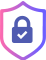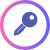# Encrypted Learning

Start to work with the data you need without having to decrypt it.## Encrypted learning is a machine learning technique that trains an algorithm on datawithout decrypting it. Data scientists can therefore use encrypted data, that was previously unworkable, to improve model accuracy.

Below, we answer an important question: “How is it possible to learn from encrypted data?”## How it works

The simple answer is that while we can’t read the data that is encrypted, we can modify it. This is best understood with a few examples.

Consider a normal algorithm like a + b = c.

If you input 2 and 3 the function will produce 5; 2 + 3 = 5.

Now consider a similar encrypted function like enc(a) + enc(b) = enc(c).

If you input enc(2) and enc(3) the function will produce enc(5); enc(2) + enc(3) = enc(5).

### Encrypted Learning allows us to add and multiply numbers together without decrypting them to produce an output.

aX + b = YA linear regression is an addition and a matrix multiplication.

becomes

enc(a) . enc(X) + enc(b) = enc(Y)An example of an encrypted linear regression.The resulting output remains encrypted and can only be decrypted by someone with the key.You can train your machine learning model and make predictions on encrypted data without exposing or reading the data.

The interesting thing is that we were able to add two numbers together without decrypting them to produce an output. The resulting output remains encrypted and can only be decrypted by someone with the key. The person with the key can now decrypt the output to reveal that the answer is 5.In machine learning, models are algorithms like the function above.

This same approach can be applied to more complex functions and models.

A linear regression is an addition and a matrix multiplication; aX + b = Y.

An encrypted linear regression would look like enc(a) . enc(X) + enc(b) = enc(Y).

Running an encrypted linear regression model on encrypted data produces the encrypted enc(Y) result. Encrypted Learning enables you to train machine learning models and/or make predictions on encrypted data without exposing or reading the data. Using this technique, data scientists can use previously inaccessible data to improve the accuracy of their model.